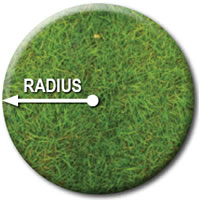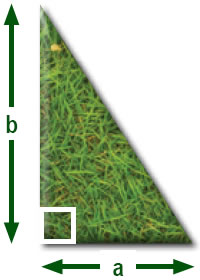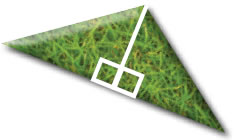# Calculator - Measuring Guide - Turf.

#### Rectangle & Square

What units are you measuring in:Finding the area in a rectangle is simple.
Multiply the short side by the long side.
No fancy tricks, just length times width.
If a rectangle was 4 feet wide and 5 feet long it would be 20 square feet.
Its the same with the square area, multiply the two sides.

Rectangle = Length x Width   Square = Length x Width

Length = Width =

Total =   Sq Meters

#### Circles

What units are you measuring in:For a circle take the radius (that's half the distance across the middle of the circle) and square it.
If the circle is 20 feet across take half of that (10) and multiply it by its self.

Circle's Area = 3.14 x Radius²
For example: 10 x 10 = 100, or 10² = 100, you would now multiply it with 3.14,
which is 100 x 3.14 = 314, therefore the area of a 20 foot circle would be 314 square feet.

Radius =

Total =   Sq Meters

#### Triangles

What units are you measuring in:Right-Angled Triangles are kind of like rectangles, taking calculations form a right angle multiply a x b then divide by 2.
This only works with RIGHT-ANGLED triangles.
That means that one corner has to be square (90º).
Triangle's Area = (a x b) ÷ 2
For example: a = 4 and b = 6, then your calculations would be 4 x 6 = 24
The area of the triangle is half of 24, 24 ÷ 2 = 12

A =   B =

Total =   Sq MetersIf your triangle isn't right-angled you need to find the area of an irregular triangle and cut it into two pieces.
Start at the corner opposite the longest side.
Go straight towards the long side, making two right-angled triangles.
Now find the area for each one of the little triangles.
Add them together to get the total area of the triangle.

#### Irregular Shapes

If your area is of an unusual shape, draw a sketch of it and then by using the four shapes above you can break it up into smaller shapes and calculate from there!

If you are unsure, please don't hesitate to contact us for advise Tel: 01294 822005.